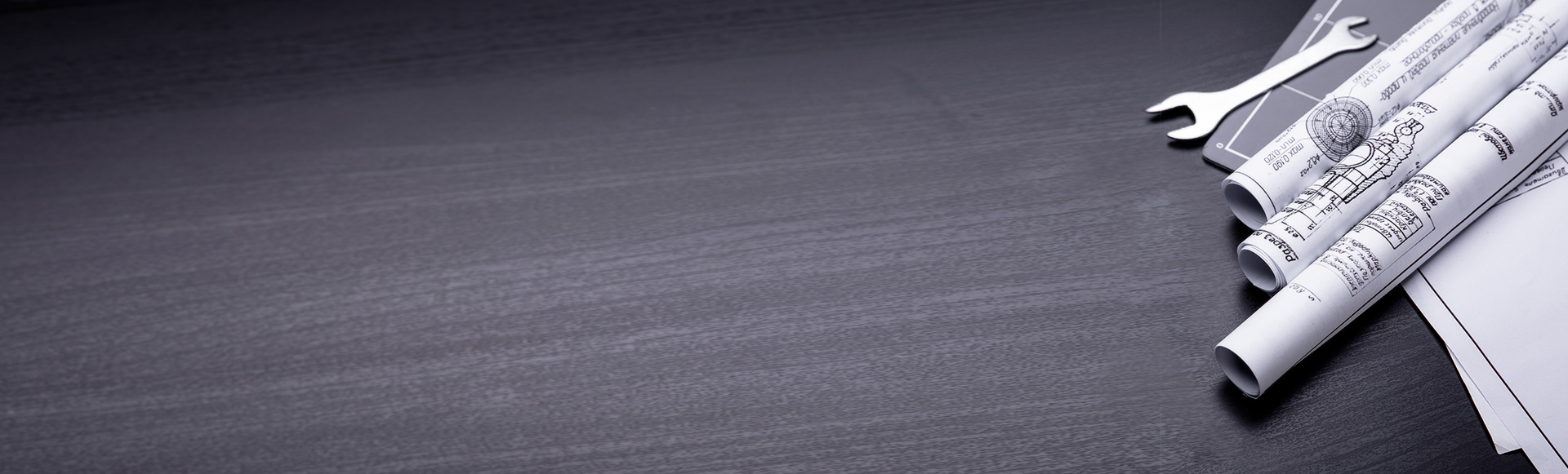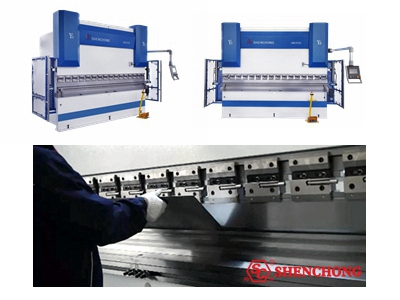MACHINE GUIDE
Position：
How to Guide: Press Brake Bending Unfolded Length Calculation [Y and K factors ]
Time：2022-09-01
Visits：526

Bending allowance can be used to calculate the unfolded length of a flat sheet metal, which for bending to be a specific radius and angle. The calculation takes into account the thickness of the sheet metal, the bending radius, the bending angle and other material properties (such as Y and K factors).

The bending allowance calculation also compensates for stretching in the bending area. When bending or forming sheet metal workpiece, the material outside bending area is usually stretched, and the material inside bending area is usually compressed. By considering the appropriate material description and material characteristic, build a formula for accurately calculating the unfolded length.

## 1. How to Calculate the Unfolded Length?### - Calculation Methods

Use one of the following methods to calculate the unfolded length in the design

- System default equation:

Calculate the unfolding length only by Y or K factors.

- Provided bend table:

Use predefined, standard bend table to calculate the unfolded length.

- Customized bend table:

Use the customized bend table in Pro/Table to calculate the unfolded length.

 L = (Π/2 x R + Y factor x T)+Θ/90 L: Unfolded length Π: 3.145 R: Inner radius Y factor: Default Y factor = 0.5 T: Material thickness Θ: Bending angle in degrees °

Note: If the unfolded length calculation is not correct, we can directly modify this value or put the unique bend table to the design to override this value.

### - Y and K Factors in Unfolded Length Calculation

Regarding the system default equation---only Y or K factors are used to calculate the unfolded length:

- The Y and K factors are workpiece constants defined by the position of the sheet metal material neutral bend line (relative to the thickness). The position of the neutral bend line is based on the numerical reference of the sheet metal material type. The numerical reference ranges from 0 to 1. If the Y and K factors are quoted, the numerical reference can be a negative number. The smaller the number, the softer the material.

- In design, the Y and K factors are necessary elements for calculating the unfolded length (the length of a flat sheet metal part required to bend the specific radius and angle). However, the length of the neutral line is equal to the expanded length.

- The K factor is the ratio between the distance from the neutral bending line to the inner press brake bending radius and the material thickness.

The calculation formula of K factor is:  K factor = δ/T

The Y factor is determined by the K factor.

- The Y factor is the ratio of the neutral bend line to the material thickness.

The calculation formula of Y factor is Y factor=K factor* (Π/2).

The default value of the Y factor is 0.50.

 The unfolded length of the material and the Y & K fact 1. Bending conditions 2. Leveling conditionsAmong them:δ= the distance between the inner bending radiusand the neutral bend line T = sheet metal thicknessL = unfolded length between squaresR = inside bending radiusN = neutral bend line K factor =δ/T Y factor =K factor * (Π/2)

## 2. How to Change the Y Factor?

We can change the Y factor by the following ways:

- Set up command:

Use the set up command to initialize the Y factor.

- The new Y factor value is valid for any new parts or features created after setting its value.

Except for those features that use user-defined Y and K factors, all parts use the default Y factor value (ie 0.5).

- Material file:

Use the PTC_INITIAL_BEND_Y_FACTOR parameter in the Material Definition dialog box, or Edit> Bend Allow> Y factor (Y – Factor) Initialize the Y factor.

- The default PTC_INITIAL_BEND_Y_FACTOR value in the material table is 0.5.

If we change the value specified for the part in the material file, the Y factor will also be updated.

- Configuration option:

Use the PTC_INITIAL_BEND_Y_FACTOR configuration option to initialize the Y factor of the new part. After reloading the configuration file, all new sheet metal parts use the new values. The configuration option does not change the default value of the Y factor of the existing part.

Get Press Brake Machine Price

We can use the feature-specific Y factor to the geometry, can select K and Y factors for non-arc line segments, and can select bend tables for arc-shaped segments. The flange profile can be arc-shaped or any non-arc-shaped section, or a combination of the two.

TAGS：
Other Columns